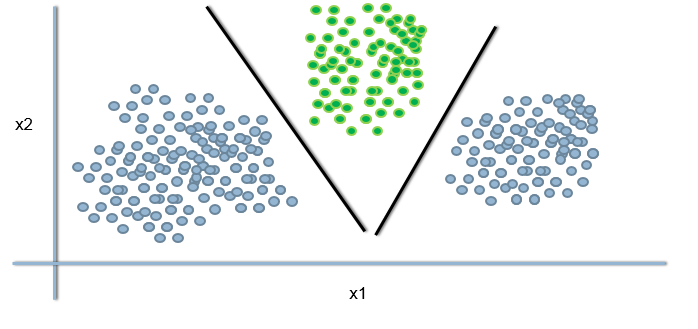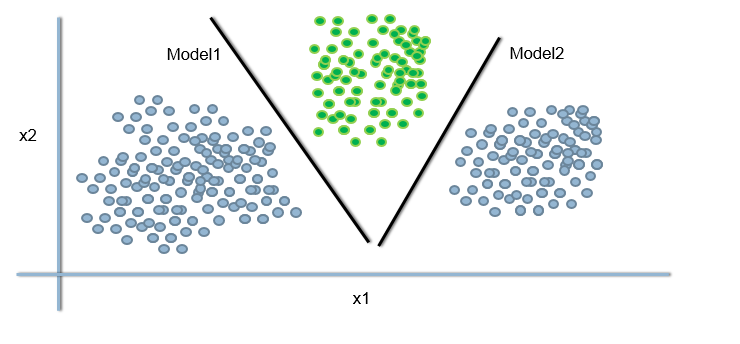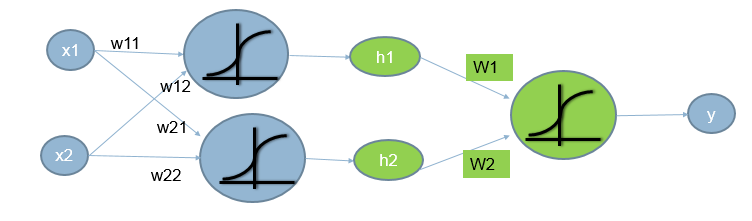• No products in the cart.

203.5.4 Issue with Non Linear Decision Boundary

Understanding intermediate outputs.

In previous post we just tried solving a Non linear Data using Linear Boundary. We can use multiple linear boundaries to separate classes in this kind of datasets.

However, this will cause some issues which we will understand bu visualizing the problem and think about a possible solution too.

Non-Linear Decision Boundaries-Issue

Logistic Regression line doesn’t seem to be a good option when we have non-linear decision boundaries.Non-Linear Decision Boundaries

Non-Linear Decision Boundaries-SolutionIntermediate Output1 Intermediate Output2
out(x)= $$g(\sum w_kx_k)$$ say h1 out(x)= $$g(\sum w_kx_k)$$ say h2

The Intermediate output

• Using the x’s directly; predicting y is challenging.
• We can predict h, the intermediate output, which will indeed predict YIf we increase the number of intermediate outputs and add a few layers of intermediate models, we end up with a basic neural network.

The next post is about a practice session on Implementing Intermediate outputs in R.

0 responses on "203.5.4 Issue with Non Linear Decision Boundary"